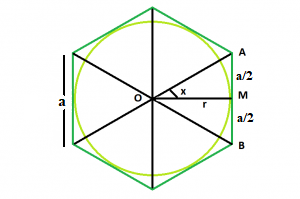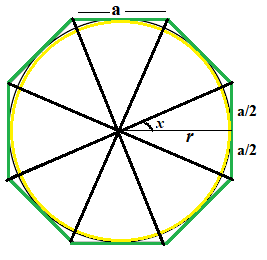Open in App
Not now

# Area of largest Circle inscribe in N-sided Regular polygon

• Last Updated : 09 Jun, 2022

Given a regular polygon of N sides with side length a. The task is to find the area of the Circle which inscribed in the polygon.
Note : This problem is mixed version of This and This
Examples:

```Input: N = 6, a = 4
Output: 37.6801
Explanation:``````In this, the polygon have 6 faces
and as we see in fig.1 we clearly see
that the angle  x  is 30 degree
so the radius of circle will be ( a / (2  * tan(30)))
Therefore, r = a√3/2

Input: N = 8, a = 8
Output: 292.81
Explanation:``````In this, the polygon have 8 faces
and as we see in fig.2 we clearly see
that the angle  x  is 22.5 degree
so the radius of circle will be ( a / (2  * tan(22.5)))
Therefore, r = a/0.828```

Approach: In the figure above, we see the polygon can be divided into N equal triangles. Looking into one of the triangles, we see that the whole angle at the center can be divided into = 360/N
So, angle x = 180/n
Now, tan(x) = (a / 2) * r
So, r = a / ( 2 * tan(x))
So, Area of the Inscribed Circle is,

` A = Πr² = Π * (a / (2 * tan(x))) * (a / (2*tan(x)))`

Below is the implementation of the above approach:

## C++

 `// C++ Program to find the area of a circle in` `// inscribed in polygon`   `#include ` `using` `namespace` `std;`   `// Function to find the area` `// of a circle` `float` `InscribedCircleArea(``float` `n, ``float` `a)` `{` `    ``// Side and side length cannot be negative` `    ``if` `(a < 0 && n < 0)` `        ``return` `-1;`   `    ``// degree converted to radians` `    ``float` `r = a / (2 * ``tan``((180 / n) * 3.14159 / 180));`   `    ``// area of circle` `    ``float` `Area = (3.14) * (r) * (r);`   `    ``return` `Area;` `}`   `// Driver code` `int` `main()` `{`   `    ``// no.  of sides` `    ``float` `n = 6;`   `    ``// side length` `    ``float` `a = 4;`   `    ``cout << InscribedCircleArea(n, a) << endl;`   `    ``return` `0;` `}`

## Java

 `// Java Program to find the area of a circle` `// inscribed in a polygon` `import` `java.io.*;`   `class` `GFG {`   `    ``// Function to find the area` `    ``// of a regular polygon` `    ``static` `float` `InscribedCircleArea(``float` `n, ``float` `a)` `    ``{` `        ``// Side and side length cannot be negative` `        ``if` `(a < ``0` `&& n < ``0``)` `            ``return` `-``1``;`   `        ``// degree converted to radians` `        ``float` `r = a / (``float``)(``2` `* Math.tan((``180` `/ n) * ``3.14159` `/ ``180``));`   `        ``// area of circle` `        ``float` `Area = (``float``)(``3.14``) * (r) * (r);`   `        ``return` `Area;` `    ``}`   `    ``// Driver code`   `    ``public` `static` `void` `main(String[] args)` `    ``{`   `        ``// no.  of sides` `        ``float` `n = ``6``;`   `        ``// side length` `        ``float` `a = ``4``;`   `        ``System.out.println(InscribedCircleArea(n, a));` `    ``}` `}`

## Python3

 `# Python 3 Program to find the area ` `# of a circle inscribed` `# in a polygon` `from` `math ``import` `tan`   `# Function to find the area of a ` `# circle` `def` `InscribedCircleArea(n, a):` `    ``# Side and side length cannot ` `    ``# be negative` `    ``if` `(a < ``0` `and` `n < ``0``):` `        ``return` `-``1`   `    ``# degree converted to radians` `    ``r ``=` `a``/``(``2` `*` `tan((``180` `/` `n) ``*` `3.14159` `/` `180``));`   `    ``# area of circle` `    ``Area ``=` `3.14` `*` `r ``*` `r`   `    ``return` `Area`   `# Driver code` `if` `__name__ ``=``=` `'__main__'``:` `    ``a ``=` `4` `    ``n ``=` `6`   `    ``print``(``'{0:.6}'``.``format``(InscribedCircleArea(n, a)))`   `# This code is contributed by` `# Chandan Agrawal`

## C#

 `// C# Program to find the area of a circle ` `// inscribed in a polygon ` `using` `System;`   `class` `GFG ` `{ `   `// Function to find the area ` `// of a regular polygon ` `static` `float` `InscribedCircleArea(``float` `n, ``float` `a) ` `{ ` `    ``// Side and side length cannot be negative ` `    ``if` `(a < 0 && n < 0) ` `        ``return` `-1; `   `    ``// degree converted to radians ` `    ``float` `r = a / (``float``)(2 * Math.Tan((180 / n) * ` `                                 ``3.14159 / 180)); `   `    ``// area of circle ` `    ``float` `Area = (``float``)(3.14) * (r) * (r); `   `    ``return` `Area; ` `} `   `// Driver code ` `public` `static` `void` `Main() ` `{ `   `    ``// no. of sides ` `    ``float` `n = 6; `   `    ``// side length ` `    ``float` `a = 4; `   `    ``Console.WriteLine(InscribedCircleArea(n, a)); ` `}` `} `   `// This code is contributed by Ryuga`

## PHP

 ``

## Javascript

 ``

Output:

`37.6801`

Time Complexity: O(1)
Auxiliary Space: O(1)

My Personal Notes arrow_drop_up
Related Articles